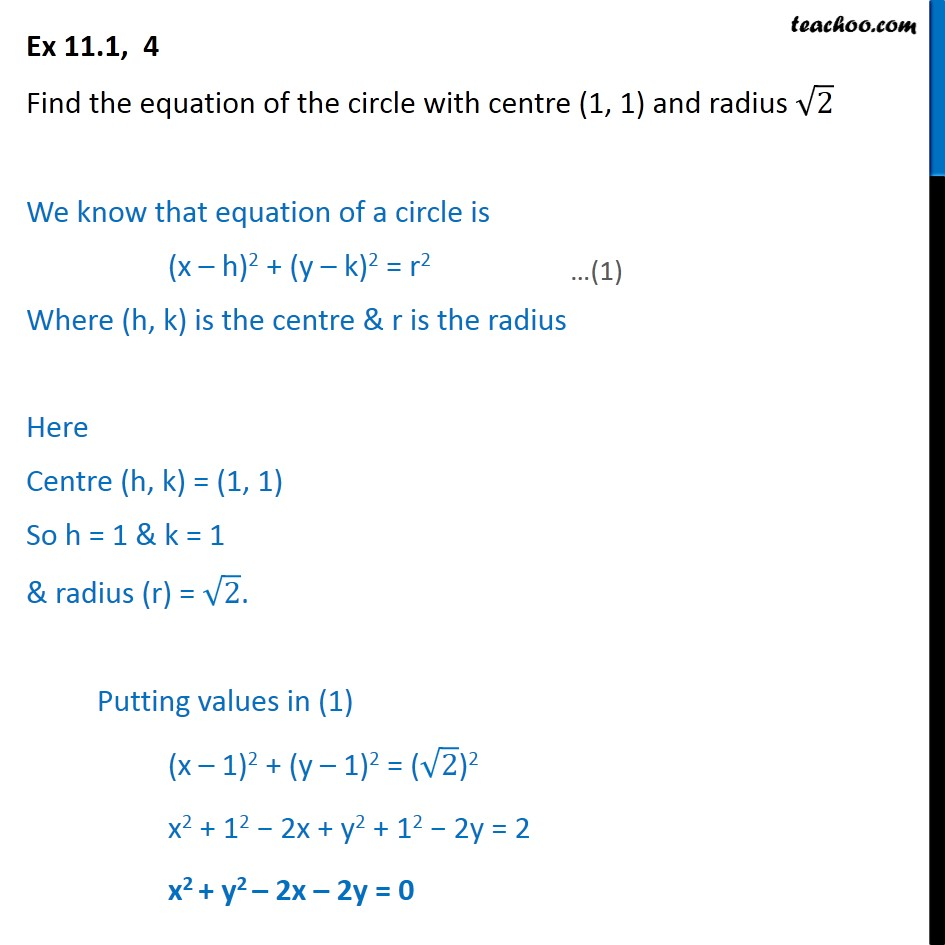Circle

Chapter 11 Class 11 Conic Sections
Concept wiseIntroducing your new favourite teacher - Teachoo Black, at only ₹83 per month

### Transcript

Ex 11.1, 4 Find the equation of the circle with centre (1, 1) and radius √2 We know that equation of a circle is (x­ – h)2 + (y ­– k)2 = r2 Where (h, k) is the centre & r is the radius Here Centre (h, k) = (1, 1) So h = 1 & k = 1 & radius (r) = √2. Putting values in (1) (x – 1)2 + (y – 1)2 = (√2)2 x2 + 12 − 2x + y2 + 12 − 2y = 2 x2 + y2 – 2x – 2y = 0